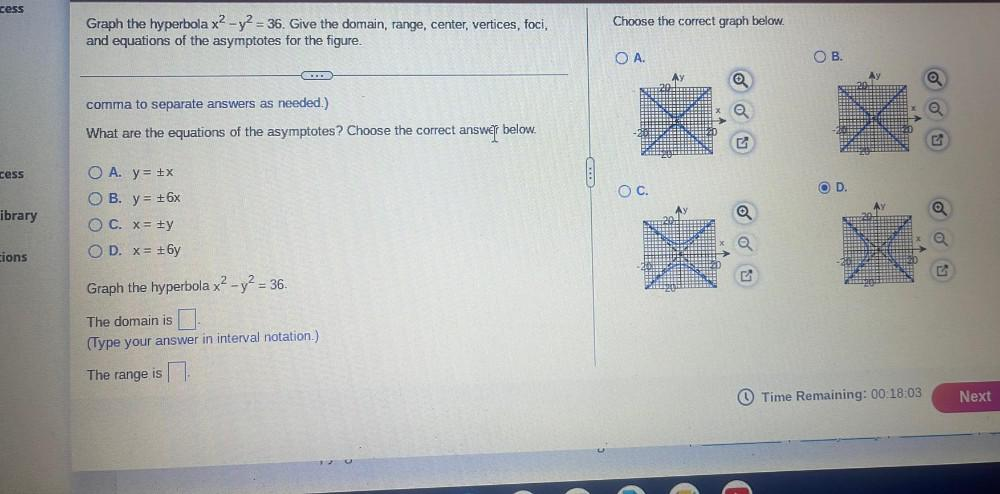Question:

# cess Choose the correct graph below. Graph the hyperbola x2 - y2 = 36. Give the domain, range, center, vertices, foci, and equatcess Choose the correct graph below. Graph the hyperbola x2 - y2 = 36. Give the domain, range, center, vertices, foci, and equations of the asymptotes for the figure. OA. OB. comma to separate answers as needed.) What are the equations of the asymptotes? Choose the correct answer below. cess OC. OD. ibrary O A. y = x O B. y= 16x OC. X= #y OD. x= +6y cions Graph the hyperbola x2 - y2 = 36. The domain is (Type your answer in interval notation.) The range is Time Remaining: 00:18:03 Next